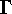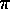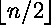# Publications (by subject, in reverse chronological order, with selected abstracts)

#### Geometric group theory

1. (with E. Berkove, S. Dharia, and R. McGuigan) The l2-cohomology of clean complexes, preprint, 2016. A clean complex is a graph of spaces whose vertex and edge spaces are graphs and whose attaching maps are embeddings. Clean complexes are notable because they provide a 2-dimensional test case for virtually special cube complexes. Given an oracle for computing in U(Fn), we present an algorithm for calculating the l2-homology of many clean complexes, including all rosy complexes (clean complexes whose underlying graphs and vertex spaces are n-leave roses). As a result, we obtain large classes of examples whose Betti numbers do not vanish and do not depend only on the Euler characteristic.
2. (with D. T. Wise) Cubulating malnormal amalgams, Invent. Math. 199 (2015), no. 2, 293-331. In this paper we examine sufficient conditions on a group G splitting as a graph of groups that ensure that G acts properly on a CAT(0) cube complex. The precise conditions are somewhat technical, but they include hyperbolicity of G relative to abelian subgroups, quasiconvexity of the vertex groups, and malnormality and subgroup separability properties of the edge groups. An additional condition involves an extension property for codimension-1 subgroups. Many of the conditions are satisfied automatically when the edge groups are free, or maximal cyclic.
3. (with D. T. Wise) Cubulating graphs of free groups with cyclic edge groups, Amer. J. Math. 132 (2010), no. 5, 1153-1188. We prove that if G is a finitely generated group that splits as a graph of free groups with cyclic edge groups, and G has no non-Euclidean Baumslag-Solitar subgroups, then G is the fundamental group of a compact nonpositively curved cube complex. In addition, if G is also word-hyperbolic (i.e., if G contains no Baumslag-Solitar subgroups of any type), we show that G is linear (in fact, is a subgroup of SLn(Z)).
4. (with I. J. Leary) Artin HNN-extensions virtually embed in Artin groups, Bull. Lon. Math. Soc. 40 (2008), no. 4, 715-719. An Artin HNN-extension is an HNN-extension of an Artin group in which the stable letter conjugates a pair of suitably chosen subsets of the standard generating set. We show that some finite index subgroup of an Artin HNN-extension embeds in an Artin group. We also obtain an analogous result for Coxeter groups. (Download at LMS)
5. (with D. T. Wise) Groups with infinitely many types of fixed subgroups, Israel J. Math. 144 (2004), 93-107. It is a theorem of Shor that if G is a word-hyperbolic group, then up to isomorphism, only finitely many groups appear as fixed subgroups of automorphisms of G. We give an example of a group G acting freely and cocompactly on a CAT(0) square complex such that infinitely many non-isomorphic groups appear as fixed subgroups of automorphisms of G. Consequently, Shor's finiteness result does not hold if the negative curvature condition is relaxed to either biautomaticity or nonpositive curvature. (pdf version) (ps version)
6. (with D. T. Wise) Ascending HNN extensions of polycyclic groups are residually finite, J. Pure Appl. Alg. 182 (2003), no. 1, 65-78. We prove that every ascending HNN extension of a polycyclic-by-finite group is residually finite. We also give a criterion for the residual finiteness of an ascending HNN extension of a residually nilpotent group, and apply this criterion to recover a result of Sapir on the residual finiteness of certain ascending HNN extensions of free groups. (pdf version) (ps version)
7. (with D. T. Wise) Separating quasiconvex subgroups of right-angled Artin groups, Math. Z. 240 (2002), no. 3, 521-548. A graph group, or right-angled Artin group, is a group given by a presentation where the only relators are commutators of the generators. A graph group presentation corresponds in a natural way to a simplicial graph, with each generator corresponding to a vertex, and each commutator relator corresponding to an edge. We show that if H is a quasiconvex subgroup of a right-angled Artin group G whose corresponding graph is a tree, then H is a separable subgroup of G, or in other words, H is the intersection of finite index subgroups of G. We also discuss some consequences relating to knot groups and 3-manifolds. (pdf version) (ps version)
8. (with D. T. Wise) On linear and residual properties of graph products, Mich. Math. J. 46 (1999), 251-259. Ifis a graph, and Gv is a set of groups indexed by the vertices of, then the graph product Gis defined to be the free product of the Gv, modulo the relations [gv,gw]=1 for all gv in Gv and gw in Gw such that (v,w) is an edge in. We show that the graph product of subgroups of Coxeter groups is a subgroup of a Coxeter group. As a result, we obtain short proofs that graph groups (right-angled Artin groups) are linear and that the graph product of residually finite groups is residually finite. We also give a new and more geometric proof of the normal form theorem for graph products. (pdf version) (ps version)
9. (with D. T. Wise) A non-residually finite square of finite groups, in C. M. Campbell et al. (eds.), Groups St. Andrews 1997 in Bath, I, volume 260 of LMS Lect. Notes, 368-378. Cambridge Univ. Press, 1999. We construct a non-positively curved non-residually finite square of finite groups whose vertex groups are of order 288, 288, 576, and 576. (In contrast, the example constructed in  has vertex groups of order between 260 and 2150.) In doing so, we demonstrate a new, more geometric method for embedding the fundamental group of a complete squared complex in the fundamental group of a square of finite groups. (pdf version) (ps version)
10. (with D. T. Wise) Embedding theorems for non-positively curved polygons of finite groups, J. Pure Appl. Alg. 123 (1998), 201-221. The fundamental groups of complete squared complexes are a class of groups, some of which are not residually finite. A method is given for embedding the fundamental group of a complete squared complex as a subgroup of a square of finite groups, all of whose (Gersten-Stallings) vertex angles are <=/2. It is also shown that every square of finite groups, all of whose vertex angles are <=/2 can be embedded in a non-positively curved triangle of finite groups. In this way, a non-residually finite, non-positively curved triangle of finite groups is obtained. (pdf version) (ps version)

#### Combinatorics of partially ordered sets

11. (with C. Petersen) Upset-downset, In preparation, 2017.
12. (with C. Day) Blue-red CHOMP, In preparation, 2017.
13. (with M. J. Logan and S. Shahriari) Methods for nesting rank 3 normalized matching rank-unimodal posets, Disc. Math. 309 (2009), no. 3, 521-531. Anderson and Griggs proved independently that a rank-symmetric-unimodal normalized matching (NM) poset possesses a nested chain decomposition (or nesting), and Griggs later conjectured that this result still holds if we remove the condition of rank-symmetry. We give several methods for constructing nestings of rank-unimodal NM posets of rank 3, which together produce substantial progress towards the rank 3 case of the Griggs nesting conjecture. In particular, we show that certain nearly symmetric posets are nested; we show that certain highly asymmetric rank 3 NM posets are nested; and we use results on minimal rank 1 NM posets to show that certain other rank 3 NM posets are nested.
14. (with M. J. Logan and S. Shahriari) The generalized Füredi conjecture holds for finite linear lattices, Disc. Math. 306 (2006), 3140-3144. We define an Anderson-Griggs poset to be a finite rank-unimodal, rank-symmetric, normalized matching poset P, and we say that P has rapidly decreasing rank numbers if below the largest ranks in the middle of P, the size of each level is at most half of the previous one. Generalizing a question of Füredi about the Boolean lattice, we conjecture that every Anderson-Griggs poset of width w has a partition into w chains such that the size of each chain is one of two consecutive integers. We prove the conjecture for all Anderson-Griggs posets with rank <= 3, and consequently obtain the conjecture for Anderson-Griggs posets with rapidly decreasing rank numbers. In particular, there exists a partition of the linear lattice Ln(q) (subspaces of an n-dimensional vector space over a field of order q, ordered by inclusion) into chains such that the number of chains is the number of subspaces of dimension, and the size of each chain is one of two consecutive integers. (pdf version) (ps version)
15. (with M. J. Logan, S. Shahriari, and C. Towse) Partitioning the Boolean lattice into a minimal number of chains of relatively uniform size, Eur. J. Comb. 24 (2003), no. 2, 219-228. Let 2[n] denote the Boolean lattice of order n, that is, the poset of subsets of {1,...,n} ordered by inclusion. Extending our previous work on a question of Füredi, we show that for any c>1, there exist functions e(n)~sqrt(n)/2 and f(n)~csqrt(nlogn) and an integer N (depending only on c) such that for all n>N, there is a chain decomposition of the Boolean lattice 2[n] intochains, all of which have size between e(n) and f(n). (A positive answer to Füredi's question would imply that the same result holds for some e(n)~sqrt(pi/2)sqrt(n) and f(n)=e(n)+1.) The main tool used is a matching property of normalized matching posets that is not hard to prove, but does not seem to have been widely used before. (pdf version) (ps version)
16. (with M. J. Logan, S. Shahriari, and C. Towse) Partitioning the Boolean lattice into chains of large minimum size, J. Comb. Thy. (A) 97 (2002), no. 1, 62-84. Let 2[n] denote the Boolean lattice of order n, that is, the poset of subsets of {1,...,n} ordered by inclusion. Recall that 2[n] may be partitioned into what we call the canonical symmetric chain decomposition (due to de Bruijn, Tengbergen, and Kruyswijk), or CSCD. Motivated by a question of Füredi, we show that there exists a function d(n)~(1/2) sqrt(n) such that for any n >= 0, 2[n] may be partitioned intochains of size at least d(n). (For comparison, a positive answer to Füredi's question would imply that the same result holds for some d(n)~sqrt(pi/2)sqrt(n).) More precisely, we first show that for 0 <= j <= n, the union of the lowest j+1 elements from each of the chains in the CSCD of 2[n] forms a poset Tj(n) with the normalized matching property and log-concave rank numbers. We then use our results on Tj(n) to show that the nodes in the CSCD chains of size less than 2d(n) may be repartitioned into chains of large minimum size, as desired. (pdf version) (ps version)

#### Expository articles

17. Rational nonaxis points on the unit circle have irrational angles, Amer. Math. Monthly 123 (2016), no. 5, 490. We give a short elementary proof that any rational point on the unit circle that does not lie on a coordinate axis has an angle that is an irrational multiple of pi.
18. (with J. H. Conway) Some very interesting sequences, in T. Shubin, D. F. Hayes, and G. Alexanderson (eds.), Expeditions in Mathematics, MAA Spectrum series, chapter 6, 75-86. MAA, Washington, DC, 2011. In this expository paper, aimed at talented high school students and mathematical enthusiasts of all ages, we discuss a few of our favorite sequences and their remarkable properties. Topics include the Fibonacci sequence, the FRACTRAN program for primes, the omnipresent Bernoulli numbers, and many others. (pdf version) (ps version)

#### Moufang loops

19. Explicit constructions of code loops as centrally twisted products, Math. Proc. Camb. Phil. Soc. 128 (2000), 223-232. Code loops are certain Moufang loop extensions of doubly even binary codes that have many applications in finite group theory (e.g., Conway's construction of the Monster). We give several methods for explicitly constructing code loops as centrally twisted products. More specifically, after establishing some preliminary examples, we show how to use decompositions of codes, such as Turyn's construction of the Golay code or the decomposition of a cyclic code into minimal cyclic codes, to build code loops out of more familiar pieces, such as abelian groups, extraspecial groups, or the octonion loop. (pdf version) (ps version)
20. Moufang loops of class 2 and cubic forms, Math. Proc. Camb. Phil. Soc. 128 (2000), 197-222. Let L be a Moufang loop that is centrally nilpotent of class 2. We first show that the nuclearly-derived subloop (normal associator subloop) L* of L has exponent dividing 6. It follows that Lp (the subloop of L of elements of p-power order) is associative for p>3. Next, a Moufang loop L is said to be a small Frattini Moufang loop, or SFML, if L has a central subgroup Z of order p such that L/Z is an elementary abelian p-group. (For instance, it follows from work of Chein and Goodaire that the SFM 2-loops are precisely the class of code loops, in the sense of Griess.) L/Z is thus given the structure of what we call a coded vector space, or CVS. (For p>2, a CVS is a vector space with attached linear, bilinear, and trilinear forms, and for p=2, a CVS is a vector space equipped with functions that act like the intersection structure of a doubly even binary code.) We show that every CVS may be obtained from an SFML in this way, and that two SFML's are isomorphic in a manner preserving the central subgroup Z if and only if their CVS's are isomorphic up to scalar multiple. Consequently, we also obtain a relatively explicit characterization of isotopy in SFM 3-loops, a characterization easily extended to Moufang loops of class 2 and exponent 3. Finally, we sketch a method for constructing any finite Moufang loop which is centrally nilpotent of class 2. (pdf version) (ps version)

#### Coset representations of modular subgroups

21. Permutation techniques for coset representations of modular subgroups, in L. Schneps (ed.), Geometric Galois Actions II: Dessins d'Enfants, Mapping Class Groups and Moduli, volume 243 of LMS Lect. Notes, 67-77. Cambridge Univ. Press, 1997. This article surveys the use of coset representations (specifying a subgroup G1<G by writing down the action of G on the cosets of G1 in G) in working with dessins d'enfants and similar structures. Questions expressed in terms of subgroups may sometimes be solved more easily in terms of coset representations. For instance, the results of  are summarized and then extended to obtain algorithms for solving problems such as finding the congruence closure of a modular subgroup. (pdf version) (ps version)
22. Identifying congruence subgroups of the modular group, Proc. AMS 124 (1996), no. 5, 1351-1359. A simple test for determining if a given subgroup of PSL2(Z) is a congruence subgroup is exhibited, and a detailed description of its implementation is given. A more "invariant" congruence test is also described. (pdf version) (ps version)

#### Quilts

23. Quilts: Central extensions, braid actions, and finite groups, volume 1731 of Lect. Notes Math., Springer-Verlag, 2000. Quilts (developed by Norton, Parker, Conway, and the author) are 2-complexes used to analyze actions and subgroups of the 3-string braid group and similar groups. This research monograph, which supersedes  and , establishes the fundamentals of quilts and discusses connections with central extensions, braid actions, and finite groups, especially in the context of the Monstrous Moonshine phenomenon. In particular, Norton's action of the 3-string braid group on pairs of elements (resp. triples of involutions) of a finite group is analyzed in detail; new results on central extensions of triangle groups, triangle group quotients, and covers of Seifert fibered 3-manifolds are discussed; several open questions from  are resolved; and some open problems are discussed. (at Springer-Verlag) (at amazon.com)
24. Quilts, the 3-string braid group, and braid actions on finite groups: an introduction, in J. Ferrar and K. Harada (eds.), The Monster and Lie Algebras, volume 7 of Ohio State Univ. Math. Res. Inst. Pubs., 85-97. de Gruyter, 1998. This article gives a brief introduction to the basic definitions and results on quilts (fully described in ). In particular, we show how quilts may be used to study Norton's action of B3 on pairs of elements (resp. triples of involutions) of a finite group, which may be relevant to the Generalized Moonshine conjectures, and we work out some small examples. (ps version)
25. Some quilts for the Mathieu groups, in C. Dong and G. Mason (eds.), Moonshine, the Monster, and Related Topics, volume 193 of Contemp. Math., 113-122. AMS, 1996. Examples of quilts arising from the Mathieu groups are presented, and some associated presentations are examined. In particular, presentations for some interesting extensions of M11, M12, and M21=L3(4) are exhibited. (ps version)
26. Quilts, T-systems, and the combinatorics of Fuchsian groups, PhD thesis, Princeton Univ., 1994.
27. (with J. H. Conway) Quilts and T-systems, J. Alg. 174 (1995), 856-908.

tim.hsu@sjsu.edu